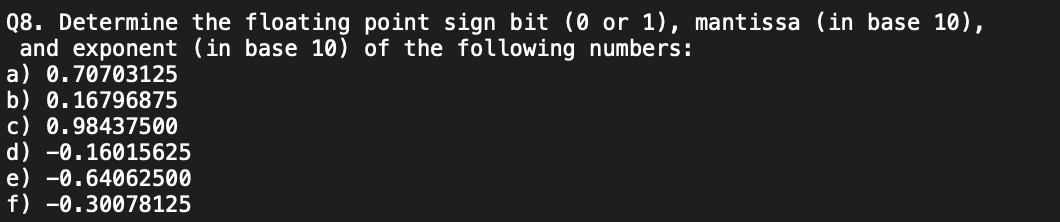Home / Expert Answers / Computer Science / q8-determine-the-floating-point-sign-bit-0-or-1-mantissa-in-base-10-and-exponent-in-base-pa928

# (Solved): Q8. Determine the floating point sign bit ( 0 or 1 ), mantissa (in base 10 ), and exponent (in base ...Q8. Determine the floating point sign bit ( 0 or 1 ), mantissa (in base 10 ), and exponent (in base 10) of the following numbers: a) 0.70703125 b) 0.16796875 c) 0.98437500 d) -0.16015625 e) -0.64062500 f) -0.30078125

We have an Answer from Expert

To determine the sign bit, mantissa, and exponent of a floating-point number, convert the given decimal numbers into binary representation following the IEEE 754 single-precision format.

The IEEE 754 single-precision format uses 32 bits, where the leftmost bit represents the sign, the next 8 bits represent the exponent, and the remaining 23 bits represent the mantissa.

Here's the step-by-step process to find the sign bit, mantissa, and exponent:

Sign Bit:
For positive numbers, the sign bit is 0.
For negative numbers, the sign bit is 1.
Convert the absolute value of the decimal number into binary representation:
To convert the decimal part, multiply the decimal number by 2 and note the integer part of the result. Repeat this process until the decimal part becomes zero or until you reach the desired precision.
To convert the integer part, repeatedly divide the number by 2 and note the remainder until the quotient becomes zero.
Determine the Exponent:
For normalized numbers, shift the binary point to the left or right until you have a single non-zero digit to the left of the binary point.
Count the number of positions you shifted the binary point. This will be the exponent.
In the IEEE 754 format, the exponent is biased by adding a bias value. For the single-precision format, the bias is 127.
Add the bias to the actual exponent to get the final exponent value.
Determine the Mantissa:
Remove the leading 1 (since it's always implied in the IEEE 754 format).
If there are more than 23 digits after the leading 1, round the digits or truncate them to fit the 23-bit mantissa.

We have an Answer from Expert